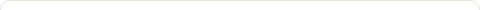# How objective and scientific are experiments?

Posted on: July 6, 2009

Article #30 of “What is Human Factors in Engineering?”;  December 27, 2005

“How objective and scientific are experiments?”

If we narrow this article to the statistical analysis of experiments and without going into details suffice us to mention a few controversies.  First, let us do a chronology of the various paradigms in statistics and statistical algorithms.  From a European perspective Pascal is believed to have started probability theory in1654.

LaPlace and Legendre contributed to the Least-Squares algorithm for how to fit a model to data (1750-1810)

Gauss developed the geometry and algebra of the multivariate normal distribution (1800’s)

Galton studied regression between two variables (1885) and Pearson the correlation coefficient in 1895.

Fisher, Snedecor and Sheffe concurrently worked on experimental design and analysis of variance algorithm (ANOVA) to statistically test the population distribution under the assumptions of normality in the 1920’s.

The data analyses of non distribution base samples to fit models to data showing structural features were developed by Thurstone in Factor Analysis, by Young and Householder (1935) in Multidimensional scaling and Cluster analysis algorithms.

Joreskog, K. G developed in 1973 the algorithm of a general method for estimating a linear structural relational equation labeled LISREL that analyses the relationships among latent variables linked to operationalized indicators. This general method considers as special cases path analysis recursive or non recursive as well as Factors analysis.

John Tukey and Mosteller concentrated on studying exploratory data analysis to fit mathematical and geometric models to data showing both structural and residual, and thus complementing confirmatory or inferential analyses.

There are divergent paradigms in the following concepts:  first, the suitability of data measurements according to measurement theory versus the distribution properties of the variable of interest (S. S. Stevens versus I. R. Savage in the 60’s); second, the need to investigate real world data prior to applying any statistical package (data snooping) so that if you perform serious detective work on the data and torture it long enough it will confess and open many ways to understand its underlying behavior (John Tukey); thus increased emphasis on graphs of individual data points and plotting to investigate the preliminary screening so as to ensure that the summary statistics selected are truly relevant to the data at hand.

Third, the application of the Bayesian approach from the consumer or decision maker viewpoint which provide the final probability against evidence instead of the investigator standard acceptance of a p-value to rejecting a hypothesis (read the “Illusion of Objectivity” by James Berger and Donald Berry, 1988).

Fourth, the selection of an investigator for a statistical package that he is familiar with instead of the appropriate statistics for the research in question;  The acceptance of untenable assumptions on population distributions and computing unrealistic parameters simply because the investigator is not trained to understanding or interpreting alternative statistical methods of nonparametric or distribution freer population methods.

Fifth, there are examples of investigators adopting explanatory statistical packages to torture data into divulging confusing causative variables while, in fact, the science is already well established in the domain to specifically determine exhaustively the causative factors simply because the investigator is not versed in mathematics or physics (“Tom Swift and his electric factor analysis machine by J. Scott Armstrong, 1967).

Sixth, there is a need to the “mathematization of behavioral sciences” (Skelum, 1969) which involves the development of mathematically stated theories leading to quantitative predictions of behavior and to derivation from the axioms of the theory of a multitude of empirically testable predictions. Thus, instead of testing verbal model as to the null hypothesis, an adequate mathematical model account for both variability and regularity in behavior and the appropriate statistical model is implied by the axioms of the model itself.  Another advantage is that attention is turned to measuring goodness of fit, range of phenomena handled by the model and ability to generating counterintuitive predictions.

This discussion is an attempt to emphasize the concept of experimentation as a structured theory and that the current easy and cheap computational potentials should be subservient to the theory so that data are transformed to answer definite and clear questions.  The Human Factors practitioner, whom should be multidisciplinary in order to master the human and physical sciences, is hard hit by the need of performing complex scientific experiments involving human subjects and yet required to yield practical recommendations for the applied engineering fields.

No wonder Human Factors professionals are confused in their purposes and ill appreciated by the other discipline unless a hybrid kind of scientists are generated from a structural combination of engineering discipline and modern experimental methods and statistical algorithms.

However, Human Factors engineers who have an undergraduate engineering discipline and a higher degree in experimental research and statistical analyses training can be better positioned to handle research involving mathematical modeling of theories in sciences.

The fixed mindedness in adolescents reminds us of the mind fix of old people with the assumption that the mind has the potential flexibility to grow while young.

You may look young masking and old mind or look older and exhibiting a younger mind; it is your choice how much time and energy you are willing to invest for acquiring knowledge.

### 1 Response to "How objective and scientific are experiments?"funny### Blog Stats

• 1,482,667 hits